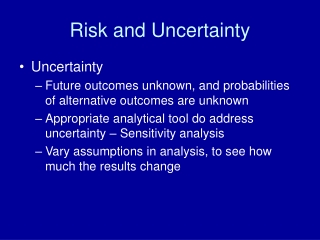DownloadDownload PresentationRisk and Uncertainty

# Risk and Uncertainty

Download Presentation## Risk and Uncertainty

- - - - - - - - - - - - - - - - - - - - - - - - - - - E N D - - - - - - - - - - - - - - - - - - - - - - - - - - -
##### Presentation Transcript

1. Risk and Uncertainty • Uncertainty • Future outcomes unknown, and probabilities of alternative outcomes are unknown • Appropriate analytical tool do address uncertainty – Sensitivity analysis • Vary assumptions in analysis, to see how much the results change

2. Analysis of Risk • Risk – Outcomes are unkown, but can estimate probabilities of different outcomes • Contingencies xi (possible States of the world) • Probabilities pi • 0 ≤ pi ≥ 1 • Σi(pi) =1 • Expected outcome (Expected Value): • Σi(pi * xi)

3. Analysis of Risk • Expected Values of Net benefits under all contingencies • Σi pi * (Bi – Ci) • Need to make sure that contingencies and associated probabilities are appropriately identified. • Spreadsheet example

4. Analysis of Risk • Projects may increase or decrease level of risk that individuals face • Risk aversion of individuals • ∂U/∂M < 0 (Diminishing Marginal utility of Money) • Compare expected utility from fair bets with certain income • Spreadsheet examples

5. Expected Utility from Fair Bet (5000 or 1000) Utility Utility Expected Utility from Fair Bet (7000 or 0) Certainty Equivalent Certainty Equivalent Income / Expected Income

6. Analysis of Risk • EU = EU{E(M) , Var(M)} • EU/E(M) > 0 • EU/Var(M) < 0

7. U2 E(M) U1 U0 Var(M)

8. Analysis of Risk • So: • Need to take into consideration effects of project on variance of income. (effect on risk) • If project increases variance of possible outcomes, this should be discounted from benefits • Some projects reduce variations of possible outcomes

9. Analysis of Risk • Example: Irrigation project • Increases expected return, but also increases variability of return • Increases probability of loss

10. Analysis of Risk • Traditional system • Cost: \$10 • Returns: 50% chance of \$12 50% chance of \$14 • Profits: 50% chance of \$2 50% chance of \$4 • Expected profit: .5 (2) + .5 (4) = \$3

11. Analysis of Risk • Irrigation system • Cost: \$30 • Returns: 50% chance of \$12 50% chance of \$80 • Profits: 50% chance of \$18 loss 50% chance of \$50 profit • Expected profit: .5 (-18) + .5 (50) = \$16

12. Analysis of Risk • Comparison of systems: • Traditional system: • Expected profit = \$3 • Variance = 1 • Irrigated system: • Expected profit = \$16 • Variance = 2,312 • AND 50% CHANCE OF LOSSES